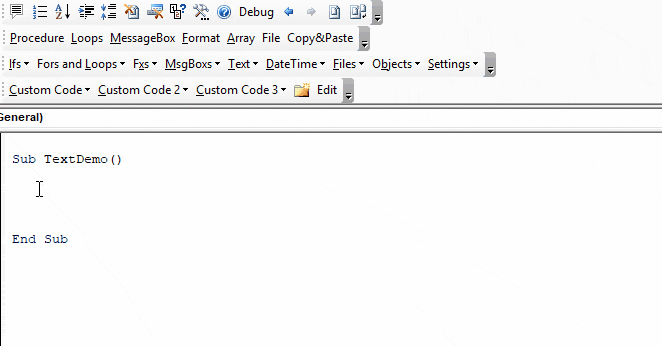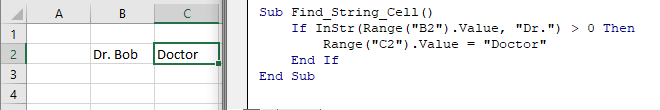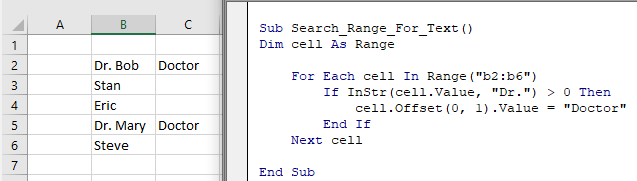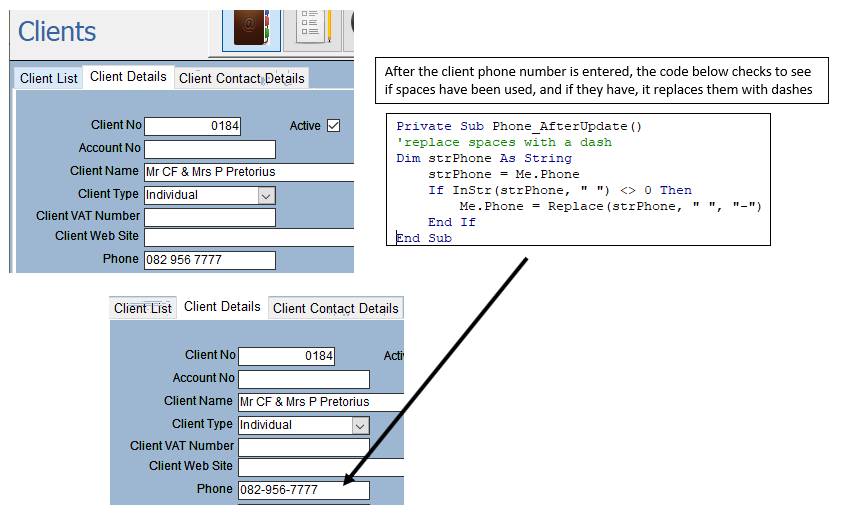# VBA INSTR – Find Text in a String

## INSTR Function

The VBA Instr Function checks if a string of text is found in another string of text.  It returns 0 if the text is not found. Otherwise it returns the character position where the text is found.

The Instr Function performs exact matches. The VBA Like Operator can be used instead to perform inexact matches / pattern matching by using Wildcards.

### Instr Example

The following code snippet searches the string “Look in this string” for the word “Look”. The Instr Function returns 1 because the text is found in the first position.

``````Sub FindSomeText()
MsgBox InStr("Look in this string", "Look")
End Sub``````

This second example returns 7 because the text is found starting in the 7th position:

``````Sub FindSomeText2()
MsgBox InStr("Don't Look in this string", "Look")
End Sub``````

Important! The Instr Function is case-sensitive by default. This means “look” will not match with “Look”. To make the test case-insensitive read below.

### Instr Syntax

The syntax for the Instr function is as follows:

``Instr( [start], string, substring, [compare] )``

[start] (optional) – This optional argument is the starting position of the search. Enter 1 to start searching from position 1 (or leave blank). Enter 5 to start searching from position 5. Important! The INSTR function calculates the character position by counting from 1 NOT from the [start] position.

string – The string of text to search in.

substring – The string of text to find in the primary string.

[compare] (optional) – By default, Instr is case-sensitive. By setting this argument you can make Instr Case insensitive:

 Argument vb Value Argument Integer Description vbBinaryCompare 0 (Default) Case-sensitive vbTextCompare 1 Not Case-sensitive vbDatabaseCompare 2 MS Access Only. Uses information in the database to perform comparison.

### Instr Start Position

The Instr start position allows you to indicate the character position where you will begin your search.  Keep in mind however, the Instr output will always count from 1.

Here we set the start position to 3 to skip the first B:

``````Sub Instr_StartPosition()
MsgBox InStr(3, "ABC ABC", "B")
End Sub``````

The result is 6 because the second B is the 6th character in the string.

### Case-Insensitive INSTR Test

By default, VBA treats “L” different from “l”. In other words, VBA is case-sensitive. This is true of all text functions.  To make VBA case-insensitive, set the [compare] argument to 1 or vbTextCompare.

``````Public Sub FindText_IgnoreCase()
MsgBox InStr(1, "Don't Look in this string", "look", vbTextCompare)
End Sub``````

Alternatively, you can add Option Compare Text to the top of your code module:

``Option Compare Text``

``````Option Compare Text

Public Sub FindText_IgnoreCase2()
MsgBox InStr("Don't Look in this string", "look")
End Sub``````

Option Compare Text will impact all of the code in that module. I personally place this at the top of any module that deals with text because I never care about case differences.

## InstrRev Function

The Instr Function searches from the left. Instead you can search from the right using the InstrRev Function.  The InstrRev Function works very similarly to the Instr function.

``````Sub FindSomeText_FromRight()
MsgBox InStrRev("Look in this string", "Look")
End Sub``````

Just like the Instr function this will return 1 because there is only one instance of “Look” in the text. But if we add a second “Look”, you’ll see that it returns the position of the right-most “Look”:

``````Sub FindSomeText_FromRight()
MsgBox InStrRev("Look in this string Look", "Look")
End Sub``````

Next we will review more Instr examples.

Stop searching for VBA code online. Learn more about AutoMacro – A VBA Code Builder that allows beginners to code procedures from scratch with minimal coding knowledge and with many time-saving features for all users!## InString Examples

### If String Contains Substring

Here we will use an If statement to test if a string contains a a substring of text:

``````Public Sub FindSomeText()

If InStr("Look in this string", "look") = 0 Then
MsgBox "No match"
Else
MsgBox "At least one match"
End If

End Sub``````

### Find Text String in a Cell

You can also find a string in a cell:

``````Sub Find_String_Cell()
If InStr(Range("B2").Value, "Dr.") > 0 Then
Range("C2").Value = "Doctor"
End If
End Sub``````Or loop through a range of cells to test if the cells contain some text:

``````Sub Search_Range_For_Text()
Dim cell As Range

For Each cell In Range("b2:b6")
If InStr(cell.Value, "Dr.") > 0 Then
cell.Offset(0, 1).Value = "Doctor"
End If
Next cell

End Sub``````VBA Programming | Code Generator does work for you!

### Find Position of a Character in a String

This code will find the position of a single character in a string and assign the position to a variable:

``````Sub Find_Char()
Dim n As Long
n = InStr("Here Look Here", "L")
End Sub``````

### Search String for Word

This code will search a string for a word:

``````Sub Search_String_For_Word()
Dim n As Long
n = InStr("Here Look Here", "Look")

If n = 0 Then
Else
MsgBox "Word found in position: " & n
End If
End Sub``````

### If Variable Contains String

This code will test if a string variable contains a string of text:

``````Sub Variable_Contains_String()
Dim str As String
str = "Look Here"

If InStr(str, "Here") > 0 Then
MsgBox "Here found!"
End If
End Sub``````

### Instr and the Left Function

Instr can be used along with other text functions like Left, Right, Len, and Mid to trim text.

With the Left function you can output the text prior to a string of text:

``````Sub Instr_Left()
Dim str As String
Dim n As Long

str = "Look Here"

n = InStr(str, "Here")

MsgBox Left(str, n - 1)

End Sub``````

## Using Instr in Microsoft Access VBA

All of the above examples work exactly the same in Access VBA as in Excel VBA.